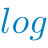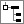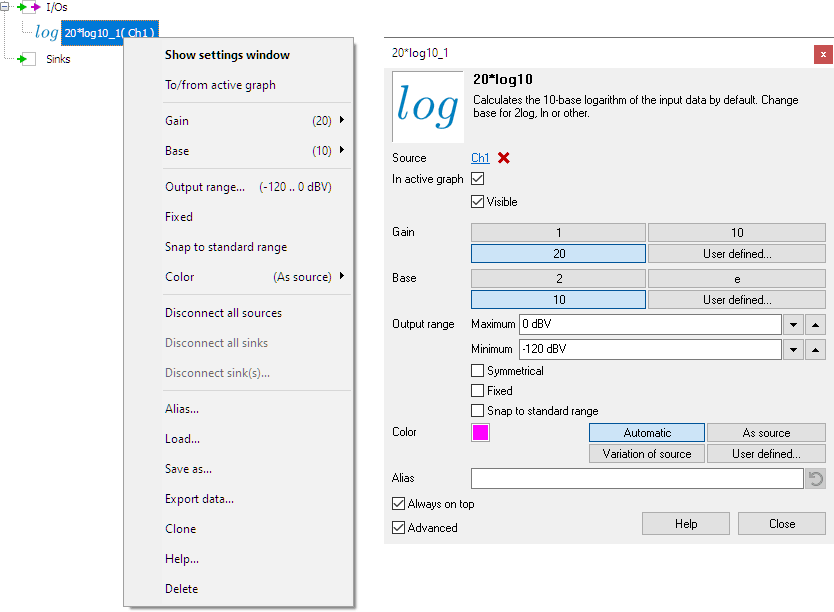# LogarithmThe Logarithm I/O calculates the logarithm of the input data. The base number and a gain can be set.

A typical application of the Logarithm I/O is to create a vertical axis in dB in the oscilloscope or spectrum analyzer.

## Properties

To control the behavior of the Logarithm I/O, several properties are available. These can be accessed through a popup menu which is shown when the I/O is right clicked in the Object Tree. The properties can also be accessed through its settings window which is shown when the I/O is double clicked in the Object Tree. To open the Object tree, click theShow object tree button.By default, the settings window only shows the most used settings. When Advanced is ticked, the extended window with all settings is shown. See also the program settings.

### Gain

The Gain property sets the gain of the Logarithm I/O. Commonly used gain factors of 1, 10 and 20 are available, as well as a user defined setting. The default value for the gain is 20.

### Base

The Base property sets the base number of the logarithm calculation. Commonly used base numbers of 2, e and 10 are available, as well as a user defined setting. The default value for the base number is 10.

### Related information

#### Gain / Offset

The Gain / Offset I/O multiplies a signal with a constant gain factor and adds a constant offset.

The Add / Subtract I/O adds or subtracts data of two or more sources.

#### Multiply / Divide

The Multiply / Divide I/O multiplies or divides data of two ore more sources.

#### Sqrt

The SQRT I/O calculates the square root of each sample of the source's data.

#### ABS

The ABS I/O takes the absolute value of each sample of the source's data.

#### Differentiate

The Differentiate I/O differentiates the source's data.

#### Integrate

The Integrate I/O integrates its source's data.